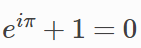# Markdown单元

LaTeX数学公式：$$e^x=\sum_{i=0}^\infty \frac{1}{i!}x^i$$


$$\int_0^{+\infty} x^2 dx$$


Jupyter notebook支持GitHub风格的语法，也就是说可以使用三个反引号和表格形式，如下：

python
print "Hello World"


javascript
console.log("Hello World")


| This | is   |
|------|------|
|   a  | table|